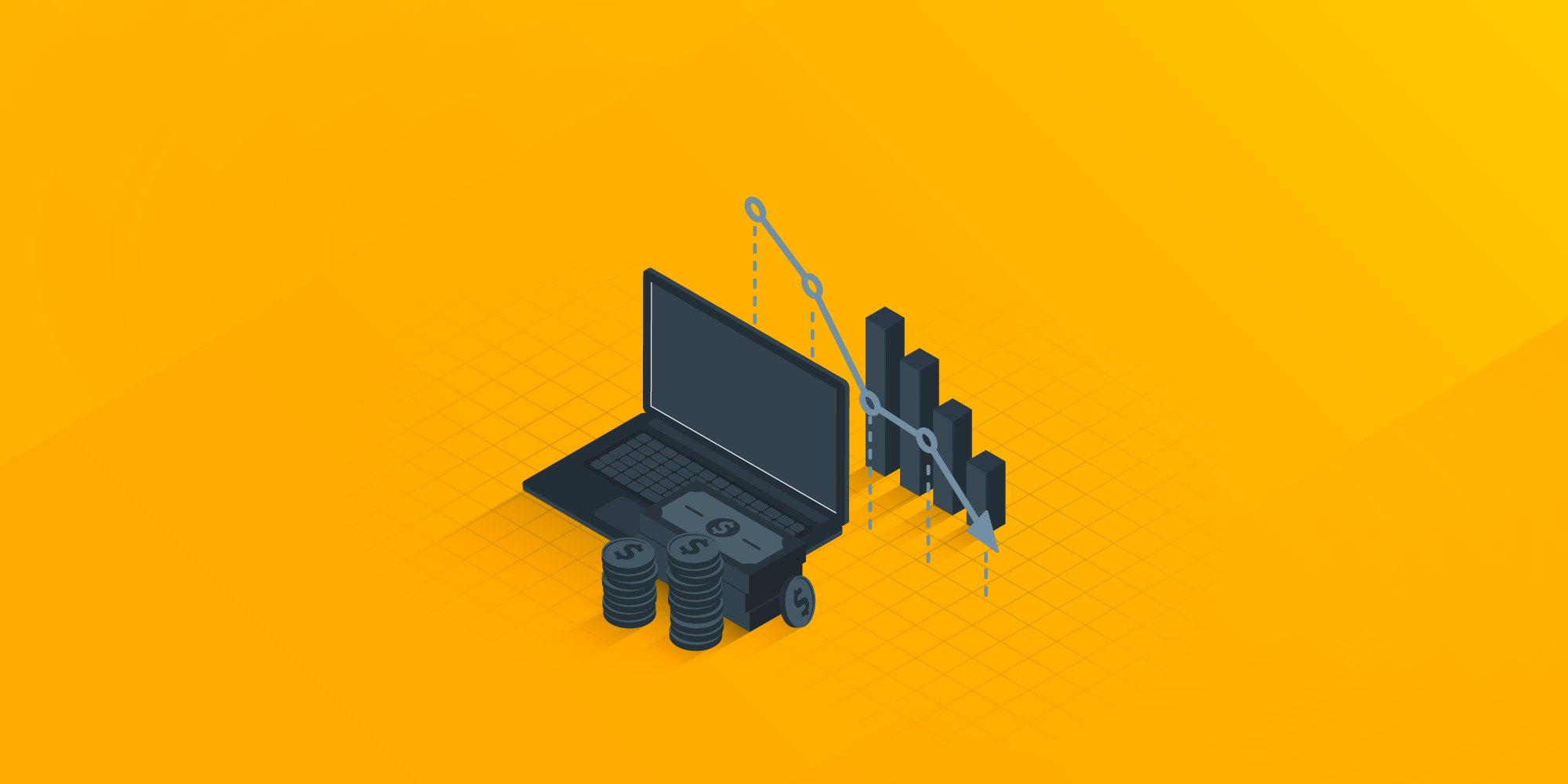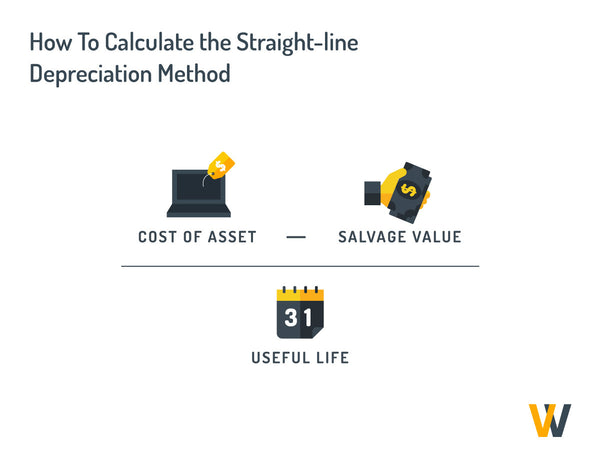# Understanding Asset Depreciation: What Is It and How To Calculate It

A business's bottom line can be affected by many factors, including asset depreciation. Understanding asset depreciation, what it is, and how to calculate it is crucial for businesses.

Buildings, vehicles, and large machinery are common assets on-site businesses use. In the remote environment, organizations focus on assets like computers or software.

Effective IT asset management for remote companies will consider depreciation to ensure that an asset's declining value over time is accounted for.

Assets are at the core of business operations since they allow you to generate revenue. Understanding the depreciation process can help businesses generate revenue or reduce taxable income in the long run.

## What is an Asset?

An asset is an item with economic value that a corporation or an individual owns with the expectation that it will provide some kind of benefit in the future. They can be classified as current, fixed, financial, and intangible.

Assets are specifically bought to create or increase an organization’s value by generating cash flow, reducing expenses, or improving sales. They can range from buildings and manufacturing equipment to patents and trademarks.

## What is Depreciation?

Depreciation is the accounting method of allocating the cost of a physical or tangible asset over its useful life. This is important because it allows businesses to account for the gradual loss of value of their assets over time and helps reduce tax liabilities.

This method allows companies to spread the cost of assets over time and reduce the immediate cost of ownership, which can impact company profit. For example, when a company buys equipment for \$40,000, it can write off the asset's price that year and report a lower profit. The other option is to write off its value over an expected lifetime of 10 years and see less impact on yearly profit.

When calculating depreciation, there are three key factors to consider:

### Useful life:

It’s the amount of time a firm expects an asset to last in terms of productivity. Depreciation will be calculated around this period.

### Salvage value:

The selling price of an asset a company uses. When businesses get rid of assets, they sell them at this salvage value. This value is helpful in depreciation to deduct it from the initial asset cost.

### Depreciation method:

There are four main types of depreciation, including the Straight-line Method, Declining Balance, Sum-of-the-Years' Digits, and Units of Production. We’ll explain each one below.

## Why is Asset Depreciation Important?

One key reason why asset depreciation is essential is that it enables businesses to report accurate financial information. Without proper depreciation, companies may overstate or understate their total asset expenses, which can lead to misleading financial information.

Another benefit of asset depreciation is that it allows companies to report a given asset's correct net book value. While most businesses report the original purchase cost of an asset, assets tend to experience wear and tear from daily use, causing their value to decline over time. By subtracting the overall depreciation expense from the original cost, companies can determine the asset's net book value, more accurately reflecting its actual value.

Furthermore, depreciation allows companies to recover the cost of an asset gradually over its lifespan rather than immediately recovering the purchase cost. This enables businesses to replace future assets using the appropriate amount of revenue, ensuring they have the necessary funds to make investments.

It's worth noting that there are tax rules that make depreciation tax deductible. A more significant depreciation expense can lower taxable income and increase tax savings, benefiting businesses looking to reduce their tax burden.

## Different Methods For Calculating Asset Depreciation

### Straight-Line DepreciationThe straight-line depreciation method is the simplest and most commonly used method for calculating asset depreciation. It involves spreading the cost of an asset evenly over its useful life.

Annual depreciation using the straight-line method is calculated by subtracting the salvage value from the asset's cost, then dividing that number by the useful life, usually expressed in years. Here’s the formula:

(Cost of Asset - Salvage Value) / Useful Life = Straight-line Depreciaiton

For example, if a company purchases a piece of machinery for \$10,000 with a useful life of 5 years and a salvage value of \$2,000, the annual depreciation expense would be: (\$10,000 - \$2,000) / 5 = \$1,600.

### Declining Balance Depreciation

The declining balance depreciation method involves calculating depreciation based on a fixed rate applied to the asset's current book value. This means that the depreciation expense will be higher in the early years of an asset's life and decrease over time.

The formula is:

Current Book Value x Depreciation Rate = Declining Balance Depreciation

For example, if a company purchases a piece of equipment for \$10,000 with a useful life of 5 years and a depreciation rate of 40%, the depreciation expense for the first year would be:

(\$10,000 x 40%) = \$4,000.

The following year, the book value would be reduced to \$6,000 (\$10,000 - \$4,000), and the depreciation expense would be calculated based on that amount.

### Sum-of-the-Years' Digits Depreciation

The sum-of-the-years digits depreciation method involves calculating depreciation based on the sum of the years of an asset's useful life. This means that the depreciation expense will be higher in the early years of an asset's life and decrease over time.

The formula is:

(Remaining Lifespan / SYD) x (Asset Cost - Salvage Value), where SYD equals the total of all the years in the lifespan.

For example, a company purchases a piece of equipment for \$10,000 and estimates to use it for a useful life of five years with a \$1,000 salvage value.

Remaining lifespan = 5

SYD = 1 + 2 + 3 + 4 + 5 = 15

SYD depreciation the first year = (5 / 15) x (\$10,000 − \$1,000)

SYD depreciation the first year = \$3,000

The company needs to subtract the depreciation expense from the book value of \$10,000 for a balance of \$7,000. Then they calculate year four in the same way, dividing the asset value by four years and continuing to do this to the first year.

### Units of Production Depreciation

Units of Production Depreciation is a method that allocates the cost of an asset over its useful life based on the number of units produced each year. This method is used when an asset's productivity can vary significantly from year to year.

The formula for Units of Production Depreciation is:

[(Asset Cost - Salvage Value) / (Estimated Total Units of production over the asset's life)] x Actual Units Produced = Units of Production Depreciation

To use this method, you will need to estimate the total number of units the asset will produce over its life and then determine the actual number of units produced each year. You can then calculate the depreciation expense by using the formula above.

This method is handy for businesses that use machinery or equipment to produce goods. It allows them to allocate the cost of the asset based on its actual usage, rather than on an arbitrary period.

## Conclusion

Asset depreciation is a vital concept in accounting and financial management. Businesses must understand the different methods of calculating asset depreciation to ensure accurate financial reporting and tax compliance.

Asset depreciation is not just an accounting practice but also an essential tool for businesses to manage their assets effectively. By depreciating assets over time, companies can account for the wear and tear of their assets and make informed decisions about when to replace or upgrade them.

For remote businesses, IT asset management solution are essential for streamlined operations regardless of locations. GroWrk can help ensure those processes occur in a single, easy-to-use platform. Give us a call today to get started.

## FAQs

### Why Are Assets Depreciated Over Time Valuable?

Asset depreciation is a valuable concept because it allows businesses to account for the wear and tear of their assets over time. This is important for accurate financial reporting and tax compliance. Depreciating assets over time also helps businesses to make informed decisions about when to replace or upgrade their assets.

### How Are Assets Depreciated For Accounting Purposes?

Assets are depreciated for accounting purposes using one of several methods, including straight-line depreciation, declining balance depreciation, sum-of-the-years' digits depreciation, and units of production depreciation. The method chosen depends on the asset's nature, useful life, and business needs.

### What Is the Difference Between Depreciation Expense and Accumulated Depreciation?

Depreciation expense is the depreciation charged against an asset in a single period, typically a year. Accumulated depreciation is the total amount a firm has depreciated its assets.

### Is Depreciation Considered To Be an Expense?

Yes, depreciation is considered an expense because it represents an asset's value loss over time. Depreciation expense is recorded on the income statement as any regular business expense.

###### Mara Quintanilla

February 27